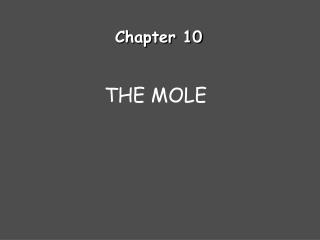DownloadDownload PresentationChapter 10

# Chapter 10

Download Presentation## Chapter 10

- - - - - - - - - - - - - - - - - - - - - - - - - - - E N D - - - - - - - - - - - - - - - - - - - - - - - - - - -
##### Presentation Transcript

1. Chapter 10 THE MOLE

3. Calculations with Moles:Converting moles to grams How many grams of lithium are in 3.50 moles of lithium? 3.50 mol Li 6.94 g Li = g Li 45.1 1 mol Li

4. Calculations with Moles:Converting grams to moles How many moles of lithium are in 18.2 grams of lithium? 18.2 g Li 1 mol Li = mol Li 2.62 6.94 g Li

5. Calculations with Moles:Using Avogadro’s Number How many atoms of lithium are in 3.50 moles of lithium? 3.50 mol Li 6.022 x 1023 atoms Li = atoms Li 2.11 x 1024 1 mol Li

6. Calculations with Moles:Using Avogadro’s Number How many atoms of lithium are in 18.2 g of lithium? 18.2 g Li 1 mol Li 6.022 x 1023 atoms Li 6.94 g Li 1 mol Li (18.2)(6.022 x 1023)/6.94 = atoms Li 1.58 x 1024

7. Calculating Molar Mass Calculate the formula mass of magnesium carbonate, MgCO3. 24.31 g + 12.01 g + 3(16.00 g) = 84.32 g

8. Calculating Percentage Composition Calculate the percentage composition of magnesium carbonate, MgCO3. From previous slide: 24.31 g + 12.01 g + 3(16.00 g) = 84.32 g 100.00

9. Formulas Empirical formula: the lowest whole number ratio of atoms in a compound. Molecular formula: the true number of atoms of each element in the formula of a compound. molecular formula = (empirical formula)n [n = integer] molecular formula = C6H6 = (CH)6 empirical formula = CH

10. Formulas(continued) Formulas for ionic compounds are ALWAYS empirical (lowest whole number ratio). Examples: NaCl MgCl2 Al2(SO4)3 K2CO3

11. Formulas(continued) Formulas for molecular compoundsMIGHT be empirical (lowest whole number ratio). Molecular: C6H12O6 H2O C12H22O11 Empirical: H2O CH2O C12H22O11

12. Empirical Formula Determination • Base calculation on 100 grams of compound. • Determine moles of each element in 100 grams of compound. • Divide each value of moles by the smallest of the values. • Multiply each number by an integer to obtain all whole numbers.

13. Empirical Formula Determination Adipic acid contains 49.32% C, 43.84% O, and 6.85% H by mass. What is the empirical formula of adipic acid?

14. Empirical Formula Determination(part 2) Divide each value of moles by the smallest of the values. Carbon: Hydrogen: Oxygen:

15. Empirical Formula Determination(part 3) Multiply each number by an integer to obtain all whole numbers. Carbon: 1.50 Hydrogen: 2.50 Oxygen: 1.00 x 2 x 2 x 2 3 5 2 C3H5O2 Empirical formula:

16. Finding the Molecular Formula The empirical formula for adipic acid is C3H5O2. The molecular mass of adipic acid is 146 g/mol. What is the molecular formula of adipic acid? 1. Find the formula mass of C3H5O2 3(12.01 g) + 5(1.01) + 2(16.00) = 73.08 g

17. Finding the Molecular Formula The empirical formula for adipic acid is C3H5O2. The molecular mass of adipic acid is 146 g/mol. What is the molecular formula of adipic acid? 2. Divide the molecular mass by the mass given by the emipirical formula. 3(12.01 g) + 5(1.01) + 2(16.00) = 73.08 g

18. Finding the Molecular Formula The empirical formula for adipic acid is C3H5O2. The molecular mass of adipic acid is 146 g/mol. What is the molecular formula of adipic acid? 3. Multiply the empirical formula by this number to get the molecular formula. 3(12.01 g) + 5(1.01) + 2(16.00) = 73.08 g (C3H5O2) x 2 = C6H10O4